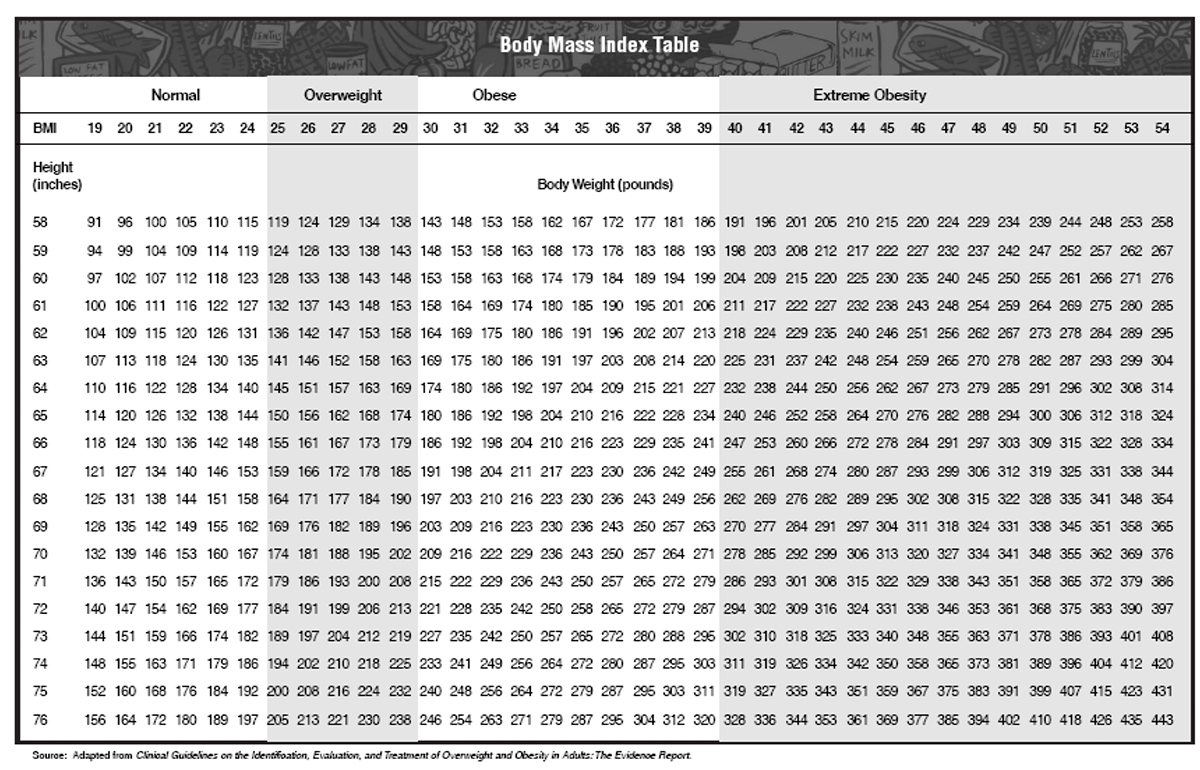Weight

Apr 2014

Guidance is compiled and interpreted by professional pilots and physicians at FlightPhysical.com from the 2014 AME Guide page 43, FAA and FDA web data (www.FAA.gov & www.FDA.gov), instructions specified in the Aeronautical Information Manual, Federal Air Surgeon Bulletins from 1999-2015, and 14 CFR Part 61 and Part 67 (the FARs).

Measure and record the applicant's weight in pounds.

BMI CHART AND FORMULA TABLE

 Units BMI Formula Pounds and inches Formula: weight (lb) / [height (in)]2 x 703 Calculate BMI by dividing weight in pounds (lbs) by height in inches (in) squared and multiplying by a conversion factor of 703. Example: Weight = 180 lbs, Height = 6'0" (72") Calculation: [180 ÷ (72)2] x 703 = 24.41 Kilograms and meters (or centimeters) Formula: weight (kg) / [height (m)]2 With the metric system, the formula for BMI is weight in kilograms divided by height in meters squared. Since height is commonly measured in centimeters, divide height in centimeters by 100 to obtain height in meters. Example: Weight = 68 kg, Height = 165 cm (1.65 m) Calculation: 68 ÷ (t (in)]2Related Pages:

The FAA is actively considering aggressive obesity screening because of the relationship with sleep apnea and excessive sleepiness which is a flight risk. The strategy is controversial and not yet policy as of March 2014.

This page discussed the Weight section of the Fight Physical Examination required of pilots.

Reminder: use FlightPhysical.com to familiarize yourself with aviation medical regulations and guidelines, but always discuss your specific situation with one or more AMEs before dedicating resources toward expensive clinical workups. Find an AME now